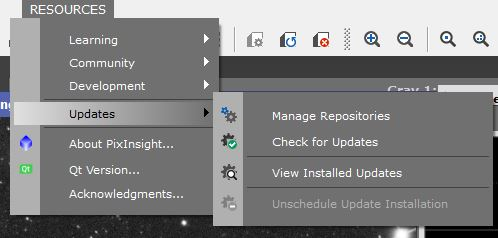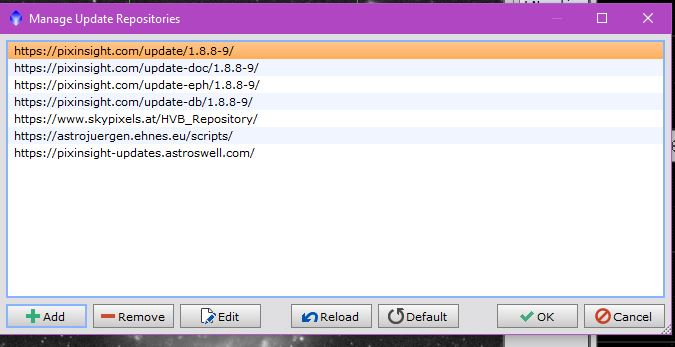January 2021 Issue!!Please click the buttons below to download any of the items you wish.

Adam Block's innovation for an Elliptical Mask with a Gradient. (He worked hard on this!)

This expression is now somewhat unnecessary (but an accomplishment for me)- in that it is now incorporated into the GAME script.

#### Expression

PA=pi()*angle/180;

r = sqrt( (((x()-offsetx)*cos(PA) + (y()-offsety)*sin(PA))/a)^2 + (((x()-offsetx)*sin(PA) - (y()-offsety)*cos(PA))/b)^2 );

iif(r<max_r, ~(r/max_r), 0)

Notes: The variable r is normalized to 1 with the a and b values controlling the scaling factors. There are other ways to do this. In the expression "r/max_r" is the linear gradient I am imposing on the resulting ellipse. Change this for a solid fill by putting a constant value (between 0 and 1) or a different function if desired. See the section on Elliptical Selections for more information.

#### Symbols

offsetx=1000,

offsety=1000,

max_r=1,

a=200,

b=100,

angle=90,

PA,

r

The values shown are arbitrary.

In PixInsight under the Resources menu option, you will find "Updates" and it is there you can manage repositories for scripts (and you will receive updates for the scripts when they happen!). To get the GAME script and others include the "skypixels" repository you see in the picture below. You are welcome to copy the others as well.Insert/Substitute Pixels (from a View/Preview) into a View

### Expression

x=x();

y=y();

x0=1095;

y0=1560;

width=870;

height=695;

iif(inrect(x0,y0,width,height),pixel(Image_To_Be_Inserted,x-x0,y-y0),\$T)

Symbols: x,y,x0,y0,width,height

The iif function is the conditional statement. Inrect is a function that checks to see if the pixel positions are within the boundaries of the defined rectangle. If yes, insert the pixel values from another view/preview. If no, do not change the target view (insert \$T).

### Symbols

x,y,x0,y0,width,height

Values here are just for an example.